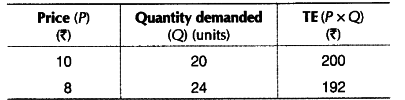# When the price of a good falls from Rs 10 to Rs 8 per unit

When the price of a good falls from Rs 10 to Rs 8 per unit, its demand rises from 20 units to 24 units. What can you say about Price Elasticity of Demand of the good through the ‘expenditure approach’ ?

Given, P = Rs 10,{{P}_{2}} = ?8, Q= 20units, Qx = 24units.As per the expenditure approach, price and TE both decreases. It shows direct relationship between price and total expenditure. So, it shows demand is inelastic or less than unity, i.e.{{E}_{d}} < I.